×#### Thank you for registering.

One of our academic counsellors will contact you within 1 working day.

Click to Chat

1800-1023-196

+91-120-4616500

CART 0

• 0

MY CART (5)

Use Coupon: CART20 and get 20% off on all online Study Material

ITEM
DETAILS
MRP
DISCOUNT
FINAL PRICE
Total Price: Rs.

There are no items in this cart.
Continue Shopping• Complete JEE Main/Advanced Course and Test Series
• OFFERED PRICE: Rs. 15,900
• View Details

```Revision Notes on Areas of Parallelograms and Triangles

Area of a Closed Shape

The part of any plane which is enclosed with the closed figure is known as the Planar Region. And the measure of this region is called the Area of that figure. This is expressed in the form of numbers using any unit.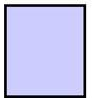Properties of the Area of a figure

If the shape and size of the two figures are the same then these are said to be congruent. And if the two figures are congruent then their area will also be the same. If ∆ABC and ∆DEF are two congruent figures then ar (∆ABC) = ar (∆DEF).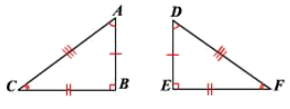But if the two figures have the same area they need not be congruent.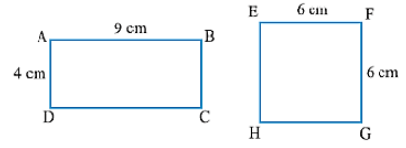If the two non-overlapping plane regions form a new planner region. Let Area of the square be ar(S) and Area of Triangle be ar (T). So Area of the new figure is Ar (P) = ar(S) + ar (T)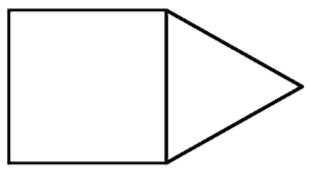Figures on the Same Base and Between the Same Parallels

If the two figures have the same base and the vertices opposite to the base is also on the line parallel to the base then the two figures are said to be on the same base and between the same parallels.∆ABC and ∆BDC have the same base and the opposite vertex is on the parallel line.

Parallelograms on the same Base and between the same Parallels

If the two parallelograms have the same base and are between the same parallel lines then these two parallelograms must have equal area.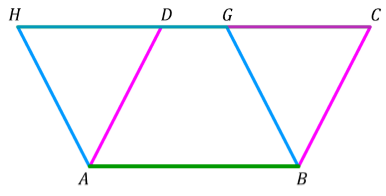Here, ABCD and ABGH are the two parallelograms having common base i.e. AB and between the two parallel lines i.e. AB and HC.

ar (ABCD) = ar (ABGH)

Remark: The parallelograms having the same base and equal area than these two parallelograms must lie between the same parallels.

Area of Parallelogram

Area of parallelogram = base × height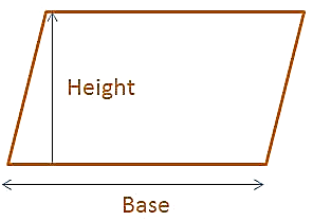Height is the perpendicular on the base.

If the Area is given and one of the height or base is missing then we can find it as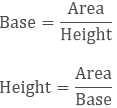Remark: The formula of area of the parallelogram is base × height that's why the two parallelograms having the same base and between the same parallel lines have equal area.

Example:

Calculate the Area of the parallelogram if the base is 15 ft and the height is 3 ft.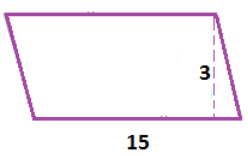Solution:

Given b = 15 ft

h = 3 ft

Area of parallelogram = b × h

= 15 × 3

= 45 ft2

Triangles on the same Base and between the same Parallels

If the two triangles are on the same base and their opposite vertex is on the parallel line then their area must be equal.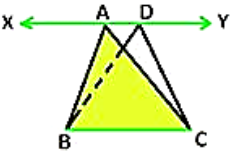Here, ABC and DBC are the two triangles having common base i.e. BC and between the two parallel lines i.e. XY and BC.

ar (ABC) = ar (DBC)

Remark: If the triangles have the same base and equal area then these two triangles must lie between the same parallels.

Area of Triangle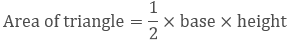Median of a Triangle

The line segment from any vertex of the triangle to the midpoint of the opposite side is the Median.

There are three medians of a triangle and the intersection of all the three medians is known as the Centroid.

The median divides the triangle into two equal parts.In ∆ABC AE, CD and BF are the three medians and the centroid is the point O.

AE divides the triangle into two equal parts i.e. ∆ACE and ∆AEB,

CD divides the triangle into two equal parts i.e. ∆CBD and ∆CDA

BF divides the triangle into two equal parts i.e. ∆BFA and ∆BFC.

A Parallelogram and a Triangle on the same base and also between same parallel

If a triangle is on the base which is same with a parallelogram and between the same parallel line then the area of the triangle is half of the area of the parallelogram.Here ∆ ABC and parallelogram ABCE are on the same base and between same parallel lines i.e. XY and BC so```### Course Features

• 728 Video Lectures
• Revision Notes
• Previous Year Papers
• Mind Map
• Study Planner
• NCERT Solutions
• Discussion Forum
• Test paper with Video Solution